# The Brown-Ecklin generator - Part 2

by W.D.Bauer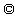1.version 15.12.96, 2. version 20.2.97, revised 3.3.97

3. Computer simulation of the Brown generator

Our model bases on one magnetic circuit, see fig.4 + 5. The source of the magnetism is a permanent magnet of constant magnetic voltage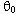. The magnetic flux due to his voltage is limited by the network of magnetic resistances in the circuit consisting of the inner magnetic resistance of the permanent magnet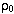, of the magnetic resistance of the stray fields, and of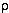the resistance of the airgap plus the rotor which consists of a rotating piece of iron in the air gap controling the magnetic flux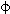. We describe it as variable resistanceor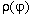dependent from position of the angle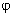during rotation. A coil in the magnetic circuit connected with a impedance of constant resistance R gives off the magnetic field energy excited by the rotating iron in the form of a time dependent electric current I(t). By this current I the coil with n windings has a magnetic voltage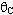=-n.I . (The sign ofhas to be minus because - as we will see later- the differential equation of the generator reduces to an usual LR-circuit under stationary conditions with=0 only if this is the case.) In order to derive the differential equation of this generator we have to find the flux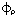at the rotor and through the coil. Therefore we analyse the network at the black dot in fig.5. At this node Kirchhoff's law holdsFor the three magnetic resistors involved Ohms law applies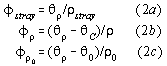From the last two equations we obtain the magnetic voltage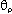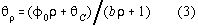whereby we used the abbreviations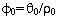and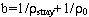. As it will be shown in the appendix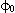can be identified with the flux through the frontal surface of the permanent magnet and b is a constant whose value depends from geometry and distance l of the airgap, if the magnetic circuit is closed by the rotor. Therefore, using (2c) and (3)becomes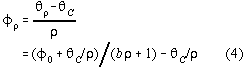Using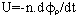, U=R.I,=-n.I and the definition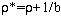the differential equation for the generator is derived toIf we add a capacitor C parallel to the resistor R (see dotted capacitor in fig.5) we would have to insert I=U/R + C.dU/dt in=-n.I and in (4) and we would obtain an differential equation of second order for the generator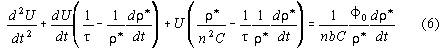where we abbreviated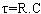.
Before we solve these equations we have to specifyand all other model parameters.contains two components which are added on in series: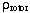is the magnetic resistance of the rotor which is neglected because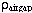-the time dependent magnetic resistance of the airgap- is much bigger. We split the last resistance again in in two parts: the constant resistanceof the remaining airgap if the rotor closes the magnetic cycle nearby at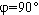in fig.4 and resistanceof rest of airgap left open additionally at other angels. If we use the formulafor the magnetic resistance of a volume of length l and area A which is filled with matter of the ferromagnetic constant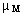, then we can estimate all values numerically. The values used in our calculation are compiled in tab.3.Becauseis small againstit is neglected and we get at frequency f(In order to facilitate iteration in our calculation the edges atof the |cos|-function were smoothed in the intervals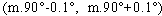by a biquadratic spline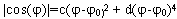. The coefficient c and d can be found assuming the function and its first derivative to be continuous.)
In order to estimate the efficiency we have to calculate the electrical and the mechanical work done after half a cycle. The electrical work output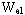at half a cycle of frequency f=1/T can be calculated toThe mechanical work input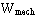is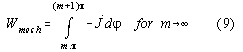whereby the torque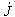for a ferromagnetic stick has been calculated to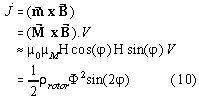In the third line we used the approximation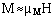, because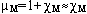for ferromagnetic materials, where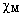:=magnetic permeability. Deriving the last line we usedand=B.A, i.e. the definitions for the magnetic resistance and magnetic flux.
Starting condition of our cycle was t=0, I=0 at 50 Hz normally. A standard Runge Kutta library routine has been used which can be found in .

Results:
Our numeric results are periodic.The initial phase starting the generator plays a role only for low load, see upper trace in fig.6. The lower trace there shows current I versus time t for a rotation frequency of 50Hz and R= 240 Ohm. The diagram is periodic for half a cycle. We see that the generator produces a pair of current spikes of opposite polarity especially at angles where the rotor closes the magnetic circuit.The first spike is higher than the second. Fig. 7 show the electric and mechanic work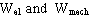versus time t at every moment measured at the resistor R at 50Hz and 240 Ohm. After half a cycle (0.01 sec) ~0.5 J are consumed by the resistor. Therefrom, we can calculate the mean electric output power of 50 W at 50 Hz delivered by the generator estimated by our calculation. The mechanic work done at this time is 2.3D-05 J. Therefrom we can estimate an efficiency 2.17D4. Fig. 8 showsversus. It is the magnetic work diagram of the coil for half a cycle and is identical to the electrical work delivered after half a cycle acc. to equ.8. It has an area of ~0.5 J. Fig.9 showsversus resistance R, fig. 10 showsversus frequency f.

Discussion:
Our model shows that overunity efficiency is possible principally from theory. We think that the tendency of our numerical results are correct surely, because a first glance at equ.6 forshows that the mechanical input work can be reduced arbitrarily choosingas high as possible without influencing the electrical output(equ.4) at all. This indicates the possibility of overunity efficiency without any numeric calculation. Furthermore, our calculation reproduces a curious behaviour described by Kromrey: The generator protects itself against being short circuited and produces less power at very low load, see fig.9. We believe that the output values are calculated in right order of magnitude. A calculation with 4 x 4 cm^2 cross section iron core resulted in 70 W output. If we realize that Brown used electromagnets for excitation whose iron cores are probably in the magnetic saturated stated to reduce back emf then it is very probable that these magnets have more than 1 T field strenght at their surface. And because output power goes with ~ I^2 or~^2 then it seem possible that his (probably RMS-) values can be correct. However, Brown's experimental input values can not be calculated correctly. This could have following causes:

1) friction:
Any friction is neglected in our model. For a real machine a input power loss P due to friction has to be added to the input. Its dependence is quadratic proportional to the rotation frequency, i.e.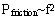. The proportional constant has to be measured or estimated according experience.

2) saturation of iron:
Magnetic material shows a saturation. This can be accounted for in the equations by makingdependent fromin our equations according to the material chosen.

3) hysteretic losses and finite transmission time of magnetism through iron:
This problem is inherent and can be reduced only by appropriated choice of magnetic material.

4) eddy currents:
Although Brown took care to avoid this problem using laminated transformer cores his setup had metallic ground plates which were electrically excited by the rotation of the machine as he proved himself. Therefore, the use of non- metallic material in the neighborhood of the machine is recommended. If possible the permanent magnets should laminated as well - or should be made out of non conducting magnetic ceramic.

5) finite time of transmission of the magnetic flux
Browns generator used laminated transformer cores, which are probably to slow for the faster harmonics of the spikes. The problem can be overcame using appropriate iron core materials.

6) bad description of the magnetic resistanceversusWe made the approximative assumption that the field across the surface of the magnet is constant. The assumption for the dependence of the magnetic resistance from angleis simple but arbitrary.
The sense of our model was to show qualitativ dependences with present data. With complete data the calculations can be improved by exact field calculation of the magnetic resistance at every angle-or- by using empirically measured values of the magnetic resistance for every. The measurement can be done by determing L at each angleand calculating the magnetic resistance acc. to the equation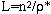which can be found by comparing coefficients of the generator equation under stationary conditions and=0 with the ordinary L/R-circuit.

Appendix: Derivation ofand b from magnet data1.) According to  the the magnetic field along the axis of an permanent magnet obeys the formula2.) If two identic cylindric magnets face one another with poles opposed, Br must be doubled at the point P.3.) Using the same geometry like 2.) but connecting the magnets with soft iron on the back L has to be replaced by 2L and Br has to be doubled.

If we short the permanent magnet(see fig.5) at the surface (by soft iron)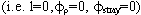then we can calculate the flux at the surface to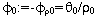acc. to equ.(2c). Using the last formula we can estimate the fluxat the surface for a cylindical neodymium magnet (R=1cm, L=2cm, Br=1,33T, l=2mm, geometry see point 2.) above ) from (A.1) and obtain=3.73D-4 Tm2. Then, the constant b can be determined using equ.(3) under stationary conditions (=0).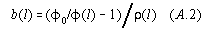At the airgap distance l=2mm (at)
we get(2mm)=5D6/(Ohm.s) and b(2mm)=4.96D-8 Vs/A .

Bibliography: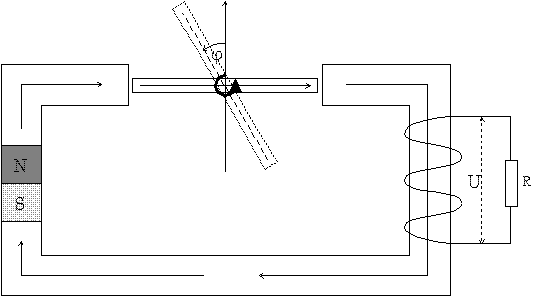Fig.4: Our model of the Brown-Ecklin generator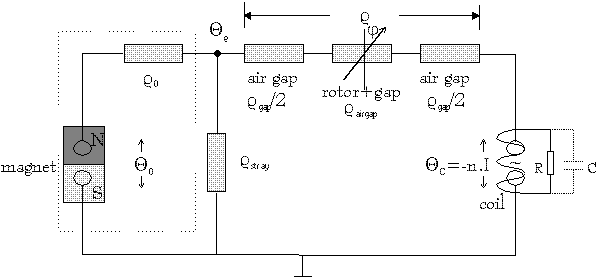Fig.5: The Brown-Ecklin generator represented as closed magnetic circuit of magnetic flux current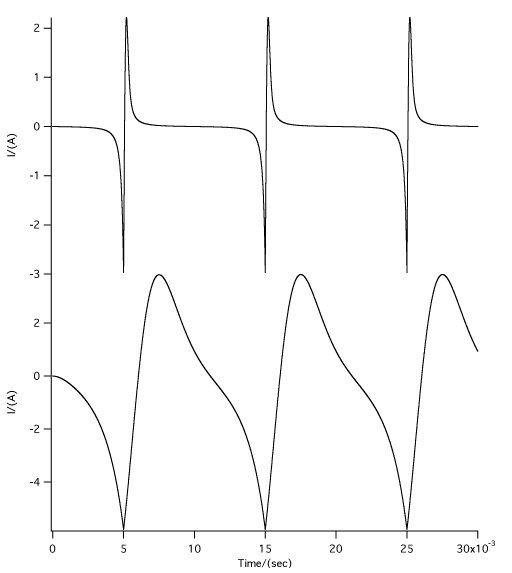Fig.6: Current I versus time of the Brown generator at 50 Hz and
lower trace 2 Ohm. upper trace 240 Ohm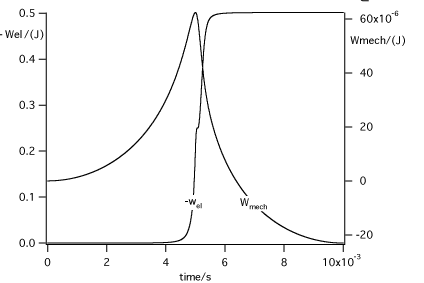Fig7: Work W vs. time t of the Brown generator at 50Hz and 240 Ohm load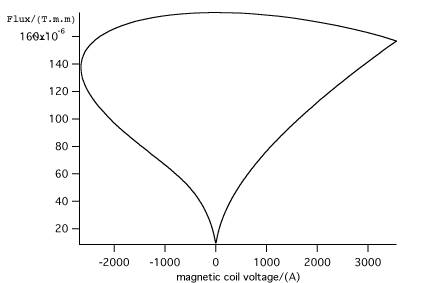Fig.8: The magnetic work diagramversusof half a cycle of 50 Hz at 240 Ohm load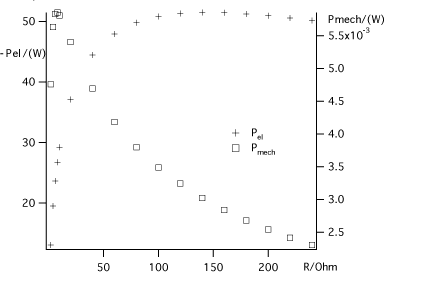Fig.9: Power P versus load R of the Brown generator at 50 Hz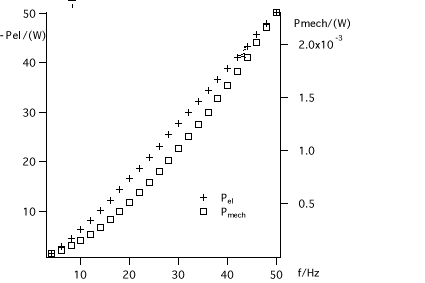Fig.10: Power P versus frequency f of the Brown generator at 240 Ohm load